• 均方误差代价函数 MSE Cost Function 代价函数可以用来衡量假设函数(hypothesis function)的准确性 在线性回归中，如果假设函数为h(x)=θ0+θ1*x, 均方误差代价函数公式如下图: θ0 和 θ1是假设函数的参数 h(x)就是...
均方误差代价函数 MSE Cost Function
代价函数可以用来衡量假设函数(hypothesis function)的准确性
在线性回归中，如果假设函数为h(x)=θ0+θ1*x, 均方误差代价函数公式如下图: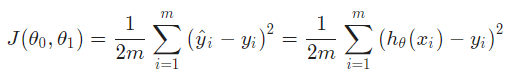θ0 和 θ1是假设函数的参数 h(x)就是预测值 m是训练的样本数量 i表示第i个样本
均方误差函数就是把预测值和真实值差的平方求和再除以2倍的样本数量
其中取2m而不取m是为了方便计算梯度.
用一道题帮助我们理解代价函数: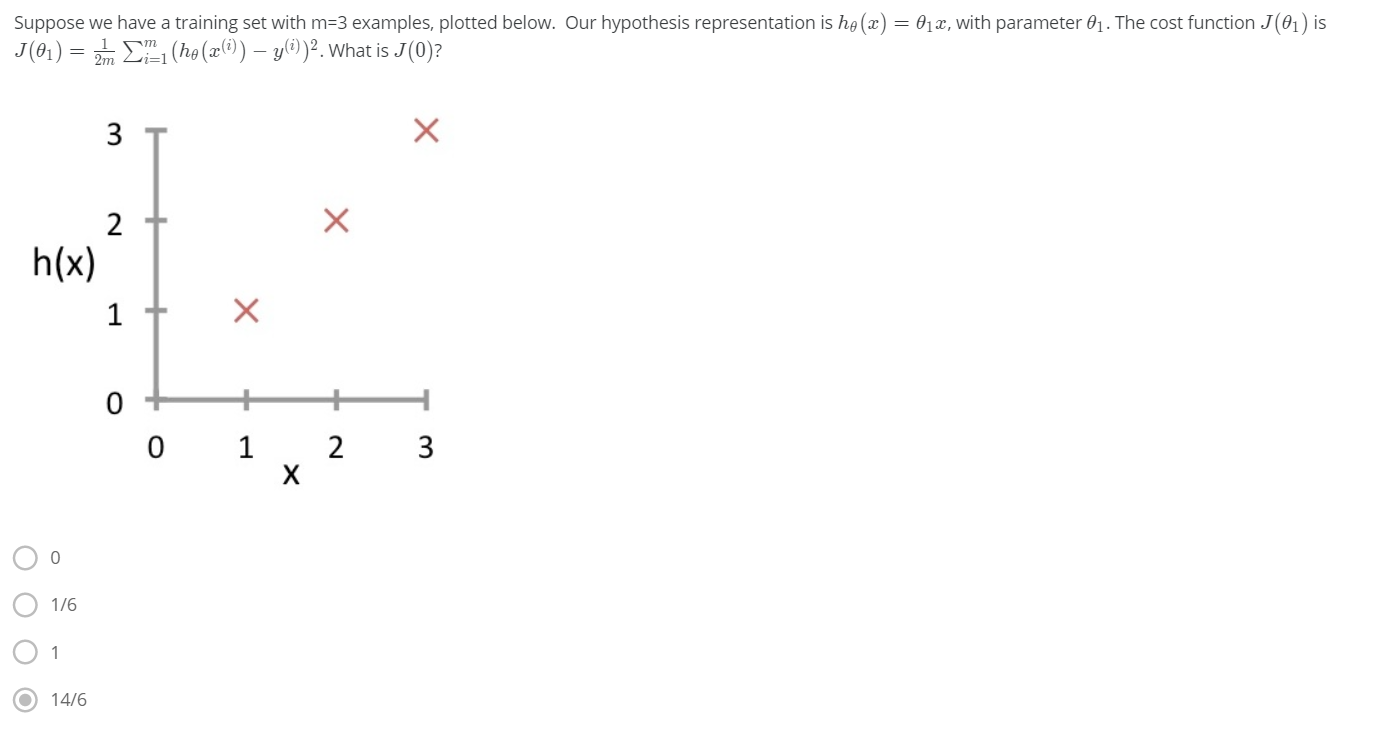这道题给了三个样本点，并且给出假设函数h(x)=θ1x,代价函数是均方误差代价函数，求J(0) 由题可知，θ0=0，要求J(0)，就是让θ1=0，当θ1=0的时候，其实h(x)=0 那么根据公式可以计算，选D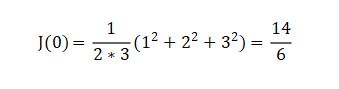当代价函数J(θ0，θ1)最小的时候，假设函数的预测值和真实值最接近 如下图，给定三个样本点(1,1),(2,2),(3,3),对于h(x)=0+θ1x,当θ1=1时，J(θ0,θ1)=0,即预测值和真实值完全相同，当θ1不为1时，预测的偏差随着θ1距离1的远近程度增加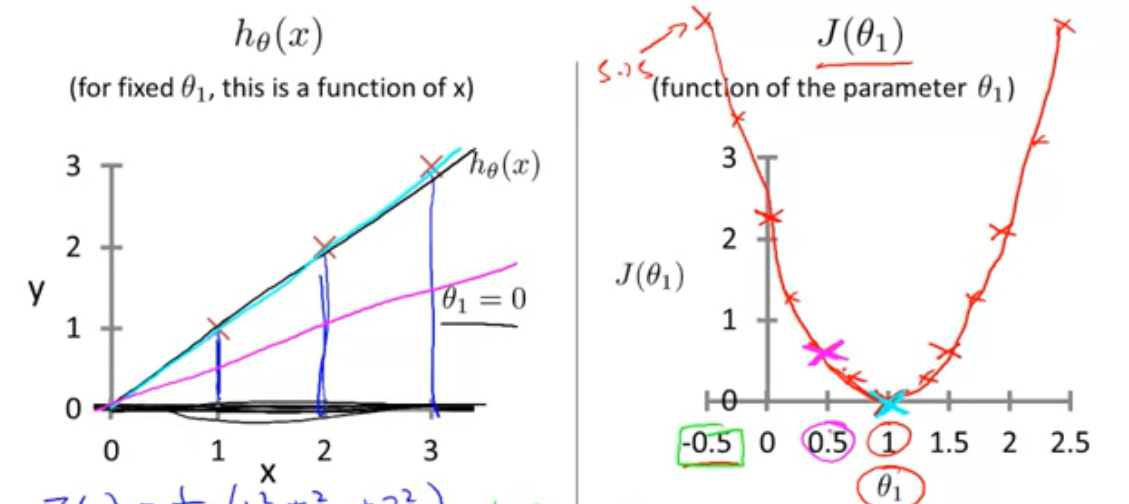展开全文机器学习
•梯度下降函数 Python实现
• 本文将从代价函数的个人理解，均方误差代数函数作用原理以及在线性回归问题中为什么选择均方误差代数函数阐述。 1、代价函数的理解： 代价函数：真实的值与预测的值之间的偏差，由于偏差有可能正有可能负，因此使用...
在机器学习中，线性回归、逻辑回归问题总是绕不开代价函数。本文将从代价函数的个人理解，均方误差代数函数作用原理以及在线性回归问题中为什么选择均方误差代数函数阐述。
1、代价函数的理解：
代价函数：真实的值与预测的值之间的偏差，由于偏差有可能正有可能负，因此使用均方差来表示。代价函数的目的即用于找到最优解。
损失函数Loss Function：定义在单个样本上，算的是一个样本的误差。 代价函数Cost Function：定义在整个训练集上，算的是所有样本的误差，也就是损失函数的平均。 目标函数Object Function：定义为最终需要优化的函数。 Object Function = Cost Function（经验风险） + 正则化项（结构风险）
2、均方误差代数函数作用原理：
对于回归问题，代价函数常选择均方误差函数。下面阐述其作用原理： 对于假设函数：

h

θ

(

x

)

=

θ

0

+

θ

1

x

h_{\theta}(x)=\theta_{0}+\theta_{1} x

参数

θ

0

\theta_{0}

和

θ

1

\theta_{1}

两个参数的变化会导致假设函数的变化: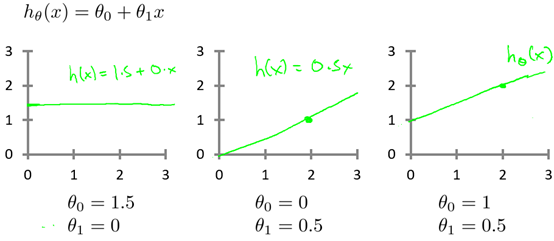实际的例子中，数据会以很多点的形式给出，回归问题就是将这些点拟合成一条曲线，即找到最优的参数

θ

0

\theta_{0}

和

θ

1

\theta_{1}

使得拟合的曲线更能代表所有的数据。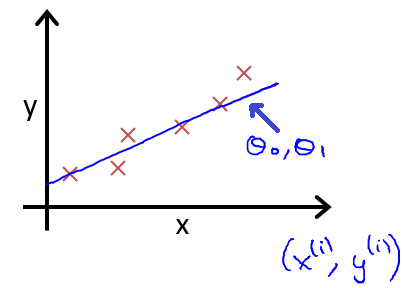如何找到最优参数

θ

0

\theta_{0}

和

θ

1

\theta_{1}

呢？使用代价函数！以均方误差代数函数为例： 从最简单的单一参数来看，假设函数为：

h

θ

(

x

)

=

θ

1

x

h_{\theta}(x)=\theta_{1} x

均方误差代数函数的主要思想就是将实际数据给出的值与拟合出的线的对应的值做差，求出拟合出的直线与实际的差距。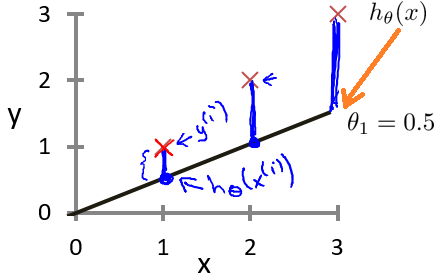为了使这个值不受个别极端数据的影响而产生巨大波动，采用类似方差再取二分之一的方式来减小个别数据影响。 这样，就产生了代价函数：

J

(

θ

0

,

θ

1

)

=

1

2

m

∑

i

=

1

m

(

h

θ

(

x

(

i

)

)

−

y

(

i

)

)

2

J\left(\theta_{0},\theta_{1}\right)=\frac{1}{2m}\sum_{i=1}^{m}\left(h_{\theta}\left(x^{(i)}\right)-y^{(i)}\right)^{2}

而最优解即为代价函数的最小值，根据以上公式多次计算可得到代价函数的图像：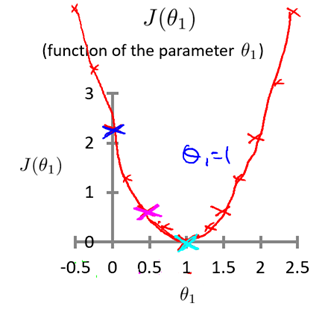可以轻易知道该代价函数的最小值(如何求解最小值？梯度下降算法~)，这时可以解出对应的 如果更多参数的话，图像就会更为复杂，两个参数的时候就已经是三维图像：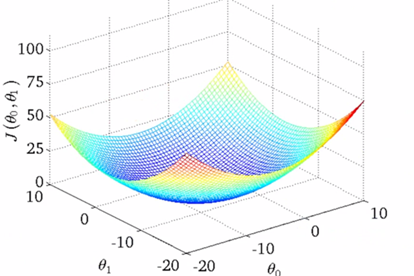高度即为均方误差代价函数的值，若参数再多则无法进行可视化，但其原理是相似的。 因此，我们可以终结得到：对于回归问题的代价函数，其最小值为：

minimize

⁡

θ

0

,

θ

1

J

(

θ

0

,

θ

1

)

\underset{\theta_{0}, \theta_{1}}{\operatorname{minimize}} J\left(\theta_{0}, \theta_{1}\right)

3、在回归问题中为什么选择均方误差函数：
从代价函数的理解考虑：代价是预测值和实际值之间的差距（两点之差），对于多个样本来说，就是差距之和。 将每一个样本的预测值和实际值之差相加，这不就是代价？但是注意到就每一个样本而言，代价有正有负，所有样本的代价加起来有可能出现正负抵消的情况，所以这并不是一个合适的代价函数（指将每一个样本的预测值和实际值之差相加）。 解决有正负的问题： 使用绝对值函数来表示代价，为了方便计算最小代价（计算最小代价可能用到最小二乘法），直接使用平方来衡量代价，即使用绝对值的平方来表示单个样本的代价，那么一个数据集的代价为：

(

h

θ

(

x

(

i

)

)

−

y

(

i

)

)

2

\left(h_{\theta}\left(x^{(i)}\right)-y^{(i)}\right)^{2}

即代价函数为：

J

(

θ

0

,

θ

1

)

=

1

2

m

∑

i

=

1

m

(

h

θ

(

x

(

i

)

)

−

y

(

i

)

)

2

J\left(\theta_{0},\theta_{1}\right)=\frac{1}{2m}\sum_{i=1}^{m}\left(h_{\theta}\left(x^{(i)}\right)-y^{(i)}\right)^{2}

为什么分母取2

m

m

而非

m

m

，是为了计算方便。
展开全文线性回归
• 均方误差函数： 交叉熵代价函数： 参考： 交叉熵代价函数(损失函数)及其求导推导
均方误差函数：交叉熵代价函数：参考：
交叉熵代价函数(损失函数)及其求导推导
展开全文• 我们设计神经网络的目的是为了实现一种模型，这个模型能够对数据做出合适的处理。比如分类问题，网络能够 以尽可能高的准确...首先，先说一下MSE，均方误差函数，这个函数在很多地方（常见于线性回归问题上，针对不...神经网络 算法
• MSE均方误差（L2 loss） 1.代码展示MAE和MSE图片特性 import tensorflow as tf import matplotlib.pyplot as plt sess = tf.Session() x_val = tf.linspace(-1.,-1.,500) target = tf.constant(0....人工智能
• 在做神经网络的训练学习过程中，一开始，经常是喜欢用二次代价函数来做损失函数，因为比较通俗易懂，后面在大部分的项目实践中却很少用到二次代价函数作为损失函数，而是用交叉熵作为损失函数。为什么？一直在思考这...
• ## 交叉熵与均方误差

千次阅读 2018-08-09 22:25:43
预期：NN在训练时，如果预测值与实际值误差越大，那么反向传播过程中，各种参数的调整的幅度就要更大，从而使训练更快收敛, 然而如果使用均方误差函数，实际有可能误差越大，参数调整的幅度可能更...机器学习 基础知识
• 损失函数 Question？ 　是什么？ 　有什么用？ 　怎么用？ 1 是什么？ 　损失函数（loss function）是用来估量你模型的预测值f(x)与真实值Y的不一致程度，它是一个非负实值函数,通常使用L(Y, f(x))来表示，...
• 在分析预滤波代价函数的基础上，首先推导经过相关后的噪声功率项表达式，然后根据最小均方误差准则，以宽度较窄的三角形单峰无模糊相关函数为赋形目标，给出包含噪声项的滤波函数最优解，最后采用预滤波器结构设计...
• 翻译 | 张建军编辑 | 阿司匹林出品 | AI科技大本营机器学习中的所有算法都依赖于最小化或最大化某一个函数，我们称之为“目标函数”。最小化的这组函数被称为“损失函数”...
• 转者注：上面虽然使用的是Sigmoid函数，其实分类问题使用的是softmax函数，softmax可以看做sigmoid在对分类问题上的推广。 转者注：上面的y1′(1−y1′)y&#x27;_1(1-y&#x27;_1)y1′​(1−y1′​)是a对z...softmax
• 方差代价函数代价函数经常用方差代价函数（即采用均方误差MSE），比如对于一个神经元（单输入单输出，sigmoid函数）,定义其代价函数为： 其中y是我们期望的输出，a为神经元的实际输出【 a=σ(z), where z=wx+b ...机器学习 深度学习
•机器学习
• 利用Tensorflow演示基于最小均差误差的线性判别函数参数拟合训练，可以作为Tensorflow卷积神经网络入门前的基础练习，练习Tensorflow的基本使用方法和模型训练的基本过程，希望对你有所帮助！ 待拟合的线性判别函数...神经网络 tensorflow 深度学习 机器学习 python
• 一。引入 　在机器学习过程中，在ppt上看到一句话，大部分的分类，回归，聚类算法，不过就是迭代，选择参数，优化目标函数。所以把代价函数整理一下。...　线性回归：用得比较多的是均方误差。 ...
• 本文是《Neural networks and ...代价函数经常用方差代价函数（即采用均方误差MSE），比如对于一个神经元（单输入单输出，sigmoid函数）,定义其代价函数为： 其中y是我们期望的输出，a为神经元的实际输出【 a=σ(z深度学习
• 代价函数经常用方差代价函数（即采用均方误差MSE），比如对于一个神经元（单输入单输出，sigmoid函数）,定义其代价函数为： 其中y是我们期望的输出，a为神经元的实际输出【 a=σ(z), where z=wx+b 】。 在训练...
• 1.代价函数 1.1 代价函数：任何可以衡量模型预测结果值与其真实值之间差异的函数，如果存在多个样本，代价... 二次代价函数(均方误差) 当A，B预测值是0.82,0.98，对应真实值为1时，A的梯度大，B的梯度小，...对数似然
• 本文主要介绍了改进神经网络的学习方式中的第一种方法，采用交叉熵函数代替均方误差函数作为代价函数。首先从反向传播的四个基本等式入手，分析出均方误差函数具有在输出错误较大时学习速度反而降低的缺点，从而引出...神经网络 深度学习
• 对于机器学习的目标，无非也就是最小化误差，也就是让代价函数最小化。训练模型的过程就是优化代价函数的过程，代价函数对每个参数的偏导数就是梯度下降中提到的梯度，防止过拟合时添加的正则化项也是...
• 代价函数（Cost Function ）是定义在整个训练集上的，是所有样本误差的平均，也就是损失函数的平均。 目标函数（Object Function）定义为：最终需要优化的函数。等于经验风险+结构风险（也就是Cost Function + 正则...机器学习 损失函数 目标函数...